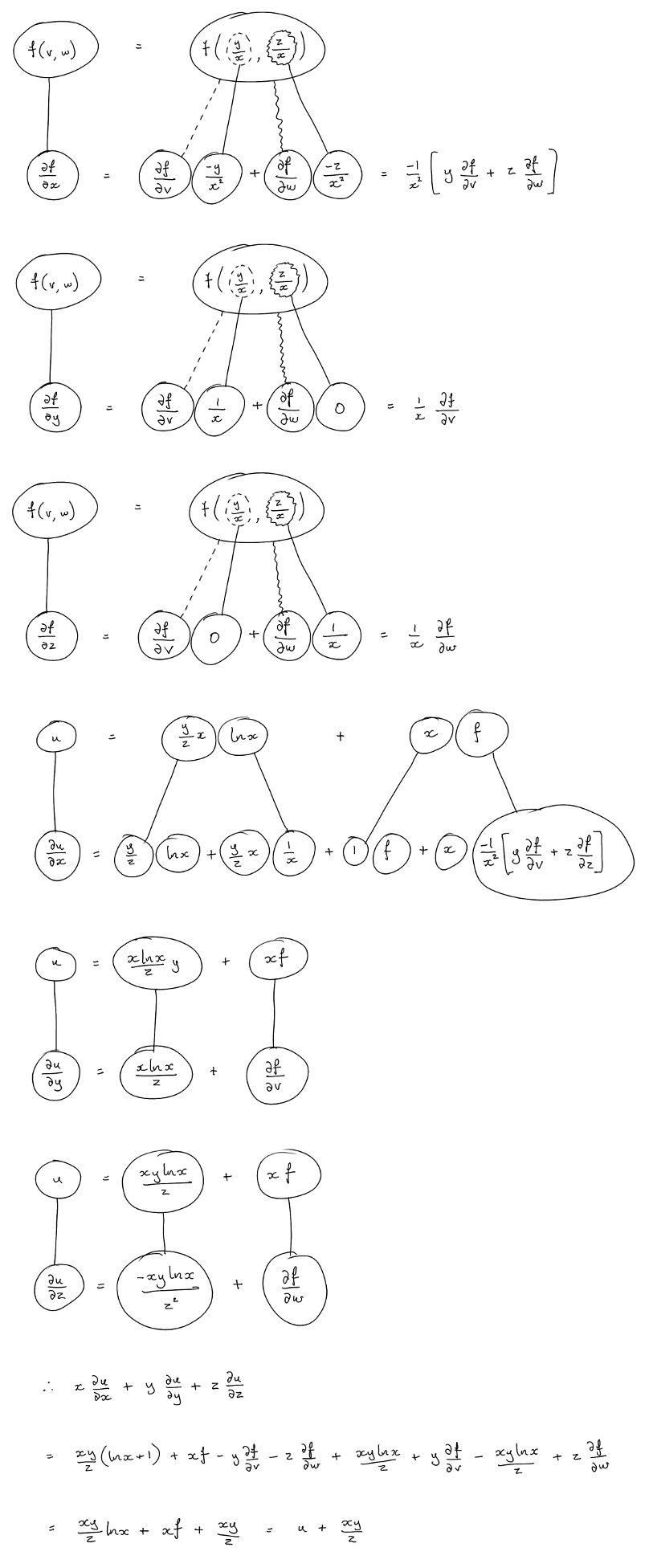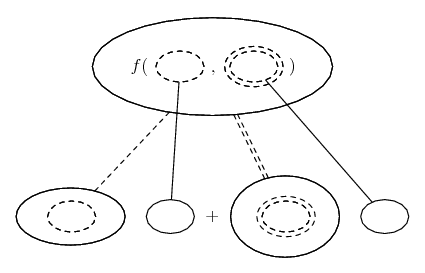# Thread: Partial derivative problem

1. ## Partial derivative problem

If $\displaystyle u=\frac{xy}{z}lnx+xf(\frac{y}{x},\frac{z}{x})$ and $\displaystyle f$ is differentiable at least once, prove that:

$\displaystyle x\frac{\partial u}{\partial x}+y\frac{\partial u}{\partial y}+z\frac{\partial u}{\partial z}=u+\frac{xy}{z}$

How to find the partial derivative with respect of x,y,z of that function f when it's not expressed.

Thanks

2. ## Re: Partial derivative problem

Just in case a picture helps...... where (key in spoiler) ...

Spoiler:is the chain rule for two inner functions, i.e...

$\displaystyle \frac{d}{dx}\ f(v(x), w(x)) = \frac{\partial f}{\partial v} \frac{dv}{dx} + \frac{\partial f}{\partial w} \frac{dw}{dx}$

As with...... the ordinary chain rule, straight continuous lines differentiate downwards (integrate up) with respect to x (or whatever), and the straight dashed line similarly but with respect to the (corresponding) dashed balloon expression which is (one of) the inner function(s) of the composite expression.

_________________________________________

Don't integrate - balloontegrate!

Balloon Calculus; standard integrals, derivatives and methods

Balloon Calculus Drawing with LaTeX and Asymptote!

#### Search Tags

derivative, partial, problem